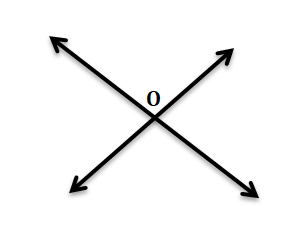Lines - Geometry
14-06-2020    71 times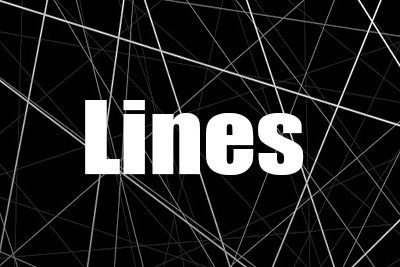### Line

When two or more points connected each other in every direction, then it constructs a line. i.e. A line is a collection of points.

line extends infinitely in two directions. The points in a same line are called collinear points. A line has length, but no breadth or thickness.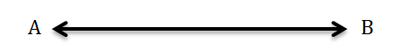Note: A line may be straight or curve but when we say ‘a line’ it means a straight line only. Each line, whatever is its length, has an infinite number of points in it.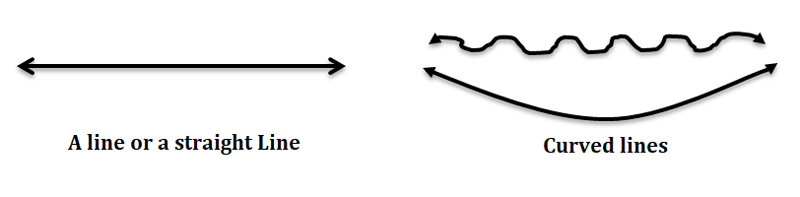A line is generally defined by two given points and is written as an arrowhead.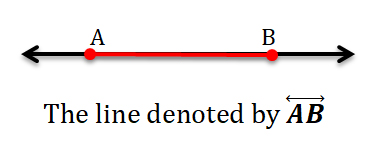### Line segment.

If a part of the line is defined by two endpoints, then that part of the line known as a line segment.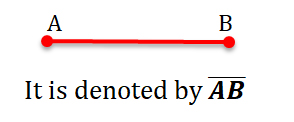### Intersecting lines and point

If two lines intersect in a point (O) then that lines are called intersecting lines and the point O known as intersecting points.